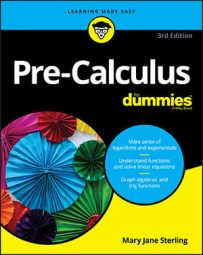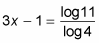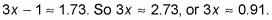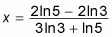##### Pre-Calculus For DummiesSometimes you just can’t express both sides of an exponential equation as powers of the same base. When facing that problem, you can make the exponent go away by taking the log of both sides.

## When the variable is on one side

For example, suppose you’re asked to solve 43x – 1 = 11. No integer with the power of 4 gives you 11, so you have to use the following technique:
1. Take the log of both sides.

You can take any log you want, but remember that you actually need to solve the equation with this log, so you should use common or natural logs only.

Using the common log on both sides gives you log 43x –1 = log 11.

2. Use the power rule to drop down the exponent.

This step gives you (3x – 1)log 4 = log 11.

3. Divide both sides by the appropriate log to isolate the variable.

You get4. Solve for the variable.

Taking the logs gives you## When you have variables on both sides

In the problem above, you had to use the power rule on only one side of the equation because the variable appeared on only one side. When you have to use the power rule on both sides, the equations can get a little messy. But with persistence, you can figure it out. For example, to solve 52 – x = 33x + 2, follow these steps:
1. Take the log of both sides.

As with the previous problem, you should use either a common log or a natural log. If you use a natural log, you get ln 52 – x = ln 33x + 2.

2. Use the power rule to drop down both exponents.

Don’t forget to include your parentheses! You get (2 – x)ln 5 = (3x + 2)ln 3.

3. Distribute the logs over the inside of the parentheses.

This step gives you 2ln 5 – xln 5 = 3xln 3 + 2ln 3.

4. Isolate the variables on one side and move everything else to the other by adding or subtracting.

You now have 2ln 5 – 2ln 3 = 3xln 3 + xln 5.

5. Factor out the x variable from all the appropriate terms.

That leaves you with 2ln 5 – 2ln 3 = x(3ln 3 + ln 5).

6. Divide the quantity in parentheses from both sides to solve for x.This equals approximately 0.208.